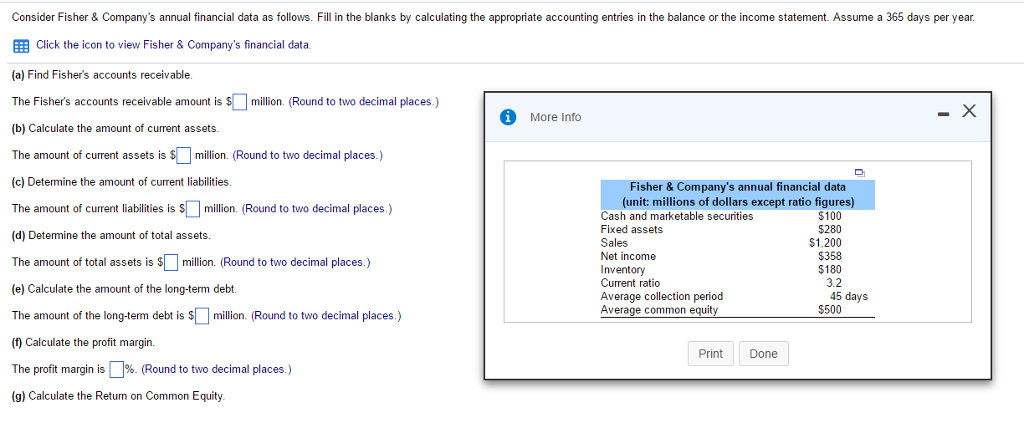# Question & Answer: Consider Fisher & Company's annual financial data as follows. Fill in the blanks by calculating the appropriate accounting entries in…..Consider Fisher & Company’s annual financial data as follows. Fill in the blanks by calculating the appropriate accounting entries in the balance or the income statement. Assume a 365 days per year. (a) Find Fisher’s accounts receivable. The Fisher’s accounts receivable amount is \$ million. (Round to two decimal places.) (b) Calculate the amount of current assets. The amount of current assets is \$ million. (Round to two decimal places.) (c) Determine the amount of current liabilities. The amount of current liabilities is \$ million. (Round to two decimal places.) (d) Determine the amount of total assets. The amount of total assets is \$ million. (Round to two decimal places.) (e) Calculate the amount of the long-term debt. The amount of the long-term debt is \$ million. (Round to two decimal places.) (f) Calculate the profit margin. The profit margin is %. (Round to two decimal places.) (g) Calculate the Return on Common Equity.

Don't use plagiarized sources. Get Your Custom Essay on
Question & Answer: Consider Fisher & Company's annual financial data as follows. Fill in the blanks by calculating the appropriate accounting entries in…..
GET AN ESSAY WRITTEN FOR YOU FROM AS LOW AS \$13/PAGE
• a) Average collection period = Accounts Receivable/ Annual Sales/365

= Accounts Receivable / \$1,200/365

= 45 days

Accounts Receivable = 45(1200 / 365) = \$147.95

b) Current assets = Cash and marketable securities + Accounts Receivable+ Inventory

= \$100 + 148 + \$180 = \$428

c) Current ratio = Current assets / Current liabilities = \$428 / Current liabilities = 3.2

Current liabilities = \$428/3.2 = \$134

d) Total assets = Currrent assets + Fixed assets = \$428 + \$280

= \$708

e) Total assets = Currrent assets + Fixed assets = \$428 + \$280 = \$708

Total assets = Common equity + Current liabilities + Long-term debt

\$708 = \$500 + \$134 + Long-term liabilities

Long-term liabilities = \$74

f) Profit-margin = Net income / Net sales = \$358 / \$1,200 = 0.298 or 30%

• g) Return on common equity = Net Income / Common equity = 358/500=71.6% or 72%